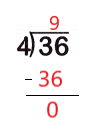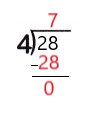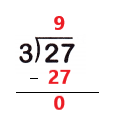# McGraw Hill Math Grade 3 Chapter 5 Lesson 8 Answer Key Dividing by 1 – 5

## McGraw-Hill Math Grade 3 Answer Key Chapter 5 Lesson 8 Dividing by 1 – 5

Solve.

Divide.

Question 1.
36 ÷ 4 = __Explanation:
36 ÷ 4 = 9
Thirty six divided by four is nine.

Question 2.
6 ÷ 3 = ___Explanation:
6 ÷ 3 = 2
Six divided by three is two.

Question 3.
15 ÷ 5 = ___
15 ÷ 5 = 3

Explanation:
I subtract 5 from 15 until i reach 0
I subtracted 5 from 15 three times
So, 15 ÷ 5 = 3.

Question 4.
14 ÷ 2 = __
14 ÷ 2 = 7

Explanation:
I subtract 2 from 14 until i reach 0
I subtracted 2 from 14 seven times
So, 14 ÷ 2 = 7.

Question 5.5 ÷ 1 = 5

Explanation:
Any number divided by 1 is the number itself
So, 5 ÷ 1 = 5.

Question 6.Explanation:
28 ÷ 4 = 7
Twenty eight divided by four is seven.

Question 7.30 ÷ 5 = 6

Explanation:
I subtract 5 from 30 until i reach 0
I subtracted 5 from 30 six times
So, 30 ÷ 5 = 6.

Question 8.Explanation:
27 ÷ 3 = 9
Twenty seven divided by three is 9.

Question 9.
Children in elementary school need about 10 hours of sleep every night. Noah slept 40 hours in 5 nights. He slept the same number of hours each night. How many hours did he sleep each night? Did he get enough sleep?